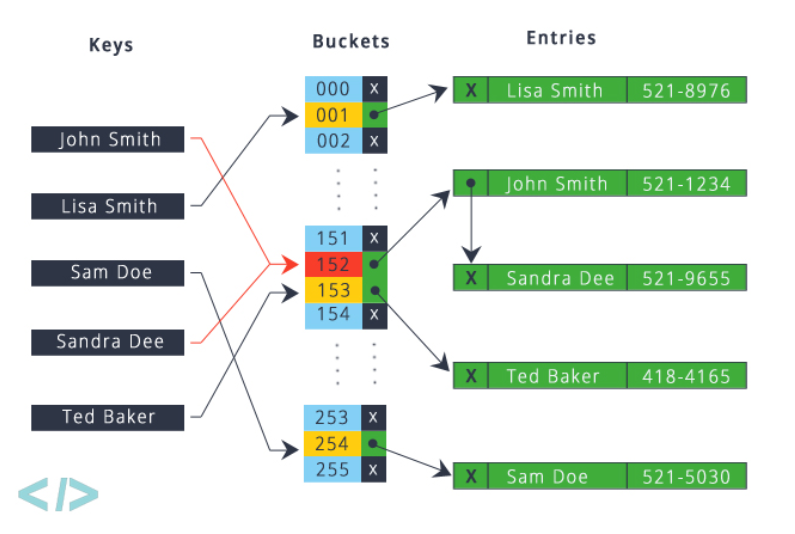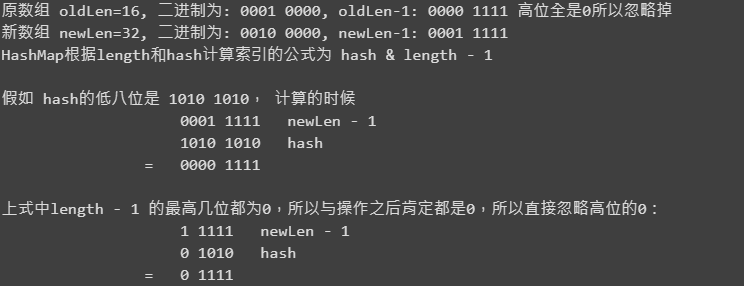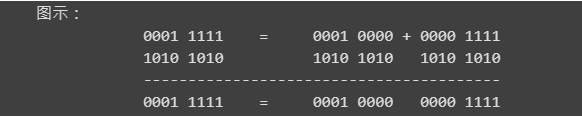##帐号 自动登录 找回密码 密码 注册

LUPA开源社区 首页 业界资讯 技术文摘 查看内容

# HashMap怎么hash？又如何map？

2018-11-2 21:51| 发布者: joejoe0332| 查看: 1072| 评论: 0|原作者: 樊腾飞|来自: oschina

HashMap 是 Java 中 Map 的一个实现类，它是一个双列结构(数据+链表)，这样的结构使得它的查询和插入效率都很高。HashMap 允许 null 键和值，它的键唯一，元素的存储无序，并且它是线程不安全的。# 双列结构：数组+链表

HashMap 的双列结构是数组 Node[]+链表，我们知道数组的查询很快，但是修改很慢，因为数组定长，所以添加或者减少元素都会导致数组扩容。而链表结构恰恰相反，它的查询慢，因为没有索引，需要遍历链表查询。但是它的修改很快，不需要扩容，只需要在首或者尾部添加即可。HashMap 正是应用了这两种数据结构，以此来保证它的查询和修改都有很高的效率。

HashMap 在调用 put() 方法存储元素的时候，会根据 key 的 hash 值来计算它的索引，这个索引有什么用呢？HashMap 使用这个索引来将这个键值对储存到对应的数组位置，比如如果计算出来的索引是 n，则它将存储在 Node[n] 这个位置。

HashMap 在计算索引的时候尽量保证它的离散，但还是会有不同的 key 计算出来的索引是一样的，那么第二次 put 的时候，key 就会产生冲突。HashMap 用链表的结构解决这个问题，当 HashMap 发现当前的索引下已经有不为 null 的 Node 存在时，会在这个 Node 后面添加新元素，同一索引下的元素就组成了链表结构，Node 和 Node 之间如何联系可以看下面 Node 类的源码分析。

DEFAULT_INITIAL_CAPACITY，默认初始长度，16：

`static final int DEFAULT_INITIAL_CAPACITY = 1 << 4; // aka 16`

MAXIMUM_CAPACITY，最大长度，2^30：

`static final int MAXIMUM_CAPACITY = 1 << 30;`

```static final float DEFAULT_LOAD_FACTOR = 0.75f;

threshold，阈值，扩容的临界值（capacity * load factor）

`int threshold;`

```// 初始长度 加载因子
public HashMap(int initialCapacity, float loadFactor)
public HashMap(int initialCapacity)

// 无参构造
public HashMap()
// 初始化一个Map
public HashMap(Map<? extends K, ? extends V> m)```

Node 类内部实现了 Map.Entry 接口中的 getKey()、getValue() 等方法，所以在遍历 Map 的时候我们可以用 Map.entrySet() 。

```static class Node<K,V> implements Map.Entry<K,V> {
final int hash; // 哈希值
final K key;
V value;
// 链表结构, 这里的next将指向链表的下一个Node键值对
Node<K,V> next;
Node(int hash, K key, V value, Node<K,V> next) {
...
}
public final K getKey()        { return key; }
public final V getValue()      { return value; }
}```

### HashMap put() 流程

put() 方法

put() 主要是将 key 和 value 保存到 Node 数组中，HashMap 根据 key 的 hash 值来确定它的索引，源码里 put 方法将调用内部的 putVal() 方法。

```public V put(K key, V value) {
return putVal(hash(key), key, value, false, true);
}```

HashMap 在 put 键值对的时候会调用 hash() 方法计算 key 的 hash 值，hash() 方法会调用 Object 的 native 方法 hashCode() 并且将计算之后的 hash 值高低位做异或运算，以增加 hash 的复杂度。（Java 里一个 int 类型占 4 个字节，一个字节是 8 bit，所以下面源码中的 h 与 h 右移 16 位就相当于高低位异或）

```static final int hash(Object key) {
int h;
return (key == null) ? 0 : (h = key.hashCode()) ^ (h >>> 16);
}
//key.hashCode() 是Object类的native方法, 实现是将内部地址转换成一个integer， 但是并不是由Java实现的
public native int hashCode();```

putVal() 方法

1. 计算容量：根据 map 的 size 计算数组容量大小，如果元素数量也就是 size 大于数组容量 ×0.75，则对数组进行扩容，扩容到原来的 2 倍。
2. 查找数据索引：根据 key 的 hash 值和数组长度找到 Node 数组索引。
3. 储存：这里有以下几种情况（假设计算出的 hash 为 i，数组为 tab，变量以代码为例）
1. 当前索引为 null，直接 new 一个 Node 并存到数组里，tab[i]=newNode(hash, key, value, null)
2. 数组不为空，这时两个元素的 hash 是一样的，再调用 equals 方法判断 key 是否一致，相同，则覆盖当前的 value，否则继续向下判断
3. 上面两个条件都不满足，说明 hash 发生冲突，Java 8 里实现了红黑树，红黑树在进行插入和删除操作时通过特定算法保持二叉查找树的平衡，从而可以获得较高的查找性能。本篇也是基于 Java 8 的源码进行分析，在这里 HashMap 会判断当前数组上的元素 tab[i] 是否是红黑树，如果是，调用红黑树的 putTreeVal 的 put 方法，它会将新元素以红黑树的数据结构储存到数组中。

```transient Node<K,V>[] table;
final V putVal(int hash, K key, V value, boolean onlyIfAbsent,
boolean evict) {
Node<K,V>[] tab; Node<K,V> p; int n, i;
// 根据当前的map size计算容量大小capacity， 主要实现是在resize()中计算capacity，需要扩容的时候， 长度左移一位（二倍）
if ((tab = table) == null || (n = tab.length) == 0)
n = (tab = resize()).length;
// 这里就是经常说的桶结构了， 看过HashMap介绍的都知道它的内部有不同的桶, 这个桶实际上就是一个链表结构
// 在这个地方， HashMap先判断key所属的桶是否存在。 (n - 1) & hash 相当于计算桶的序号， 根据桶序号来找到对应的桶
// 这里的table 是HashMap的数组， 数组为空就新建一个数组 newNode(hash, key, value, null)
if ((p = tab[i = (n - 1) & hash]) == null)
tab[i] = newNode(hash, key, value, null);
else {
//数组不为空， 先判断key是否存在， 存在 就覆盖value
Node<K,V> e; K k;
if (p.hash == hash &&
((k = p.key) == key || (key != null && key.equals(k))))
e = p;
// 如果此链表是红黑树结构（TreeNode）
else if (p instanceof TreeNode)
e = ((TreeNode<K,V>)p).putTreeVal(this, tab, hash, key, value);
else {
// 循环当前链表， 找出p.next为空的位置就是链表的末端， 添加上
for (int binCount = 0; ; ++binCount) {
if ((e = p.next) == null) {
p.next = newNode(hash, key, value, null);
// 这里会判断这个链表是否需要转换为红黑树链表
if (binCount >= TREEIFY_THRESHOLD - 1) // -1 for 1st
treeifyBin(tab, hash);
break;
}
if (e.hash == hash &&
((k = e.key) == key || (key != null && key.equals(k))))
break;
p = e;
}
}
if (e != null) { // existing mapping for key
V oldValue = e.value;
if (!onlyIfAbsent || oldValue == null)
e.value = value;
afterNodeAccess(e);
return oldValue;
}
}
++modCount;
if (++size > threshold)
// put之后，如果元素个数大于当前的数组容量了，进行数组扩容
resize();
afterNodeInsertion(evict);
return null;
}```

### HashMap 的 get()

get() 方法会调用 getNode() 方法，这是 get() 的核心，getNode() 方法的两个参数分别是 hash 值和 key。

```public V get(Object key) {
Node<K,V> e;
return (e = getNode(hash(key), key)) == null ? null : e.value;
}```

1. 根据 hash 值和数组长度找到 key 对应的数组索引。
2. 拿到当前的数组元素，也就是这个链表的第一个元素 first，先用 hash 和 equals() 判断是不是第一个元素，是的话直接返回，不是的话继续下面的逻辑。
3. 不是链表的第一个元素，判断这个元素 first 是不是红黑树，如果是调用红黑树的 getTreeNode 方法来查询。
4. 如果不是红黑树结构，从 first 元素开始遍历当前链表，直到找到要查询的元素，如果没有则返回 null。
```final Node<K,V> getNode(int hash, Object key) {
// tab： HashMap的数组
Node<K,V>[] tab;
Node<K,V> first, e;
int n; K k;
// 判断数组不为空， 桶不为空
if ((tab = table) != null && (n = tab.length) > 0 &&
(first = tab[(n - 1) & hash]) != null) {
// 先查询桶的第一个元素
if (first.hash == hash && // always check first node
((k = first.key) == key || (key != null && key.equals(k))))
return first;
// 不是第一个元素
if ((e = first.next) != null) {
// 如果是红黑树， 则用红黑树的方法查询
if (first instanceof TreeNode)
return ((TreeNode<K,V>)first).getTreeNode(hash, key);
// 不是红黑树， 遍历桶， 直到找到对应的key， 返回
do {
// 1. 判断hash值是否相等； 2. 判断key相等。 防止hash碰撞发生
if (e.hash == hash &&
((k = e.key) == key || (key != null && key.equals(k))))
return e;
} while ((e = e.next) != null);
}
}
return null;
}```

### 数组扩容时再哈希(re-hash)的理解```Node<K,V> loHead = null, loTail = null;  // 低位索引（原索引）上的元素
Node<K,V> hiHead = null, hiTail = null;  // 高位索引（新索引）上的元素
Node<K,V> next;
do {
next = e.next;
// 判断是否需要放到新的索引上
if ((e.hash & oldCap) == 0) {
// 最高非零位与操作结果是0，扩容后元素索引不发生变化
if (loTail == null)
else
loTail.next = e;
loTail = e;
}
else {
// 需要将元素放到新的索引上
if (hiTail == null)
else
hiTail.next = e;
hiTail = e;
}
} while ((e = next) != null);
if (loTail != null) {
loTail.next = null;
// 这部分的链表索引没有发生变化，将链表放到原索引上
}
if (hiTail != null) {
hiTail.next = null;
// 这部分的链表索引发生变化，将链表放到新索引上
newTab[j + oldCap] = hiHead;
}```

# HashMap 与 HashTable

• HashMap 是线程不安全的，HashTable 线程安全，因为它在 get、put 方法上加了 synchronized 关键字。
• HashMap 和 HashTable 的 hash 值是不一样的，所在的桶的计算方式也不一样。HashMap 的桶是通过 & 运算符来实现 (tab.length - 1) & hash，而 HashTable 是通过取余计算，速度更慢（hash & 0x7FFFFFFF) % tab.length （当 tab.length = 2^n 时，因为 HashMap 的数组长度正好都是 2^n，所以两者是等价的）
• HashTable 的 synchronized 是方法级别的，也就是它是在 put() 方法上加的，这也就是说任何一个 put 操作都会使用同一个锁，而实际上不同索引上的元素之间彼此操作不会受到影响；ConcurrentHashMap 相当于是 HashTable 的升级，它也是线程安全的，而且只有在同一个桶上加锁，也就是说只有在多个线程操作同一个数组索引的时候才加锁，极大提高了效率。

# 总结

• HashMap 底层是数组+链表结构，数组长度默认是 16，当元素的个数大于数组长度×0.75 时，数组会扩容。
• HashMap 是散列表，它根据 key 的 hash 值来找到对应的数组索引来储存， 发生 hash 碰撞的时候（计算出来的 hash 值相等） HashMap 将采用拉链式来储存元素，也就是我们所说的单向链表结构。
• 在 Java7 中，如果 hash 碰撞，导致拉链过长，查询的性能会下降， 所以在 Java8 中添加红黑树结构，当一个桶的长度超过 8 时，将其转为红黑树链表，如果小于 6，又重新转换为普通链表。
• re-hash 再哈希问题：HashMap 扩容的时候会重新计算每一个元素的索引，重新计算之后的索引只有两种可能，要么等于原索引要么等于原索引加上原数组长度。
• 由上一条可知，每次扩容，整个 hash table 都需要重新计算索引，非常耗时，所以在日常使用中一定要注意这个问题。

# 作者介绍